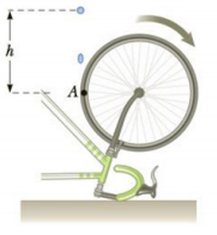Chapter 7, Problem 8P

Chapter
Section
Textbook Problem

A bicycle is turned upside down while its owner repairs a flat tire. A friend spins the other wheel and observes that drops of water fly off tangentially. She measures the heights reached by drops moving vertically (Fig. P7.8). A drop that breaks loose from the tire on one turn rises vertically 54.0 cm above the tangent point. A drop that breaks loose on the next turn rises 51.0 cm above the tangent point. The radius of the wheel is 0.381 m. (a) Why does the first drop rise higher than the second drop? (b) Neglecting air friction and using only the observed heights and the radius of the wheel, find the wheel’s angular acceleration (assuming it to be constant).Figure P7.8Problems 8 and 69.

(a)

To determine
The reason the first drop rise higher than the second drop.

Explanation

Given info: The bicycle is turned upside down. The wheel of the cycle is spinned. The drops of water on the tire fly off tangentially. The height reached by the first drop is 54.0cm which more than the height reached by the second drop that is 51.0cm . The radius of the wheel is 0.318m .

Explanation:

The water drop flying off the cycle tire can be treated like a projectile

b)

To determine
The wheel’s angular acceleration.

Still sussing out bartleby?

Check out a sample textbook solution.

See a sample solution

The Solution to Your Study Problems

Bartleby provides explanations to thousands of textbook problems written by our experts, many with advanced degrees!

Get Started

How does astronomy help answer the question “What are we?”

Horizons: Exploring the Universe (MindTap Course List)

Why are buffers important for living organisms?

Biology: The Dynamic Science (MindTap Course List)

How are atoms different from molecules?

Oceanography: An Invitation To Marine Science, Loose-leaf Versin